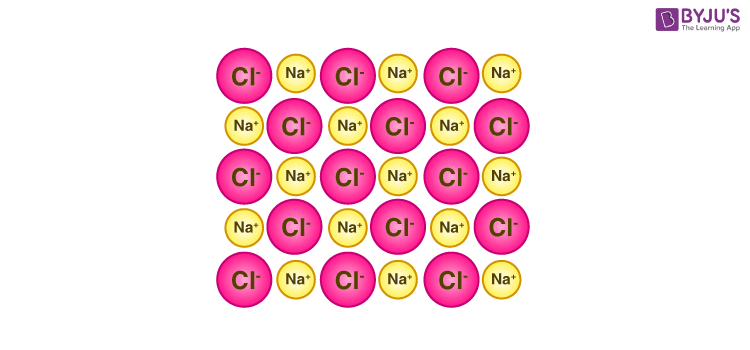# Lattice Energy

## What is Lattice Energy?

• Lattice energy is a measure of the strength of the ionic bonds in an ionic compound.
• It provides insight into several properties of ionic solids including their volatility, their solubility, and their hardness.
• The lattice energy of an ionic solid cannot be measured directly.
• However, it can be estimated with the help of the Born-Haber cycle.
• Generally, this quantity is expressed in terms of kilojoules per mole (kJ/mol).

### Definition

Lattice energy can be defined as the energy required to convert one mole of an ionic solid into gaseous ionic constituents.

• Alternatively, it can be defined as the energy that must be supplied to one mole of an ionic crystal in order to separate it into gaseous ions in a vacuum via an endothermic process.
• Therefore, this quantity always holds a positive value.
• Some sources define lattice energy in the opposite manner, i.e. the amount of energy released when an ionic solid is formed from its gaseous ionic constituents via an exothermic process.
• According to this definition, lattice energy must always hold a negative value.

### Example

The crystal lattice of a sodium chloride molecule is illustrated below.Lattice Energy in NaCl

In the case of this ionic molecule, the lattice energy is the energy required for the following reaction to proceed.

NaCl(s) → Na+ (g) + Cl (g)

Here, the energy that must be supplied to 1 mole of sodium chloride to separate it into gaseous Na+ and Cl ions is 786 kilojoules.

## Comparison Between Lattice Energy and Lattice Enthalpy

The molar lattice energy of an ionic crystal can be expressed in terms of molar lattice enthalpy, pressure, and change in volume via the following equation:

ΔLatticeU = ΔLatticeH – pΔVm

Where:

• ΔLatticeU denotes the molar lattice energy.
• ΔLatticeH denotes the molar lattice enthalpy.
• ΔVm is the change in volume (per mole).
• p is the pressure.

Therefore, the outer pressure is also considered when calculating the lattice energies of ionic solids.

## What are the factors that Affect Lattice Energy?

The two primary factors that affect the lattice energy of an ionic compound are the magnitude of charge associated with the constituent ions and the distance between the ions.

### Charge held by the Constituent Ions

Due to the electrostatic forces between them, the individual ions in an ionic lattice are attracted to each other. The strength of the electrostatic force of attraction is directly proportional to the magnitude of the charge held by the constituent ions, i.e. the greater the charge, the stronger the force of attraction, and the stronger the lattice.

For example, the lattice energy of calcium chloride is greater than that of potassium chloride despite the similarity in the crystal arrangements of these compounds. This is because the magnitude of the positive charge held by the calcium cation (+2) is greater than that held by the potassium cation (+1). As a consequence of this, the electrostatic forces of attraction are stronger in calcium chloride (than those in potassium chloride). Therefore, the lattice energy of CaCl2 is greater than that of KCl.

### Distance between the Ions

The lattice energy of an ionic compound is inversely proportional to the distance between the ions. The farther the distance between the ions in a lattice, the weaker the electrostatic forces holding them together, and the lower the lattice energy.

Smaller atoms feature smaller interatomic distances in the ionic lattice and stronger binding forces. Therefore, the smaller the size of the constituent ions, the greater the lattice energy of the ionic solid.

## Calculating Lattice Energy using the Born-Haber Cycle

The Born-Haber cycle is based on Hess’ law of constant heat of summation. According to Hess’ laws, if a chemical reaction takes place in one step or several steps the total heat of the reaction is constant. For Example, for a chemical reaction given by A → B; The heat of reaction (ΔH) = +Q.

Alternately, if the reaction takes place over several steps:

A → C ΔH1 = q1

C → D ΔH2 = q2

D → B ΔH3 = q3

Then, according to Hess’ law,  +Q = q1 + q2 + q3. It can be noted that this law holds good for cyclic processes as well.

## Solid State | Concept Visualised | Crystal Lattice and Unit Cell## Calculating the Change in Energy for the formation of NaCl (Born-Haber Cycle)

Sodium chloride can be formed via two methods:

Direct Method: Metallic sodium reacts with chlorine gas to form sodium chloride.

Indirect method: This method of forming sodium chloride involves several steps:

Sublimation of sodium: Solid sodium changes to gaseous sodium via a sublimation process. The energy required for this to occur is the sublimation energy (+S).

The ionization of sodium: The sodium atom loses an electron to form a sodium ion. The energy required is the ionization potential (+IP).

Dissociation of chlorine: The chlorine molecule dissociates into two chlorine atoms, the energy required is the dissociation energy (+D/2).

Formation of chloride ion: The Cl atom accepts an electron to form a chloride ion. The energy liberate is the electron affinity (-Ea).

Formation of Sodium Chloride: Sodium ions combine with chloride ions to form sodium chloride. The amount of energy liberated is the lattice energy.

Therefore, the value of ΔHf is equal to the sum of all the energies listed above. To learn more about lattice enthalpy and lattice energy, register with BYJU’S and download the mobile application on your smartphone.

Test your Knowledge on Lattice energy!